Courses
Courses for Kids
Free study material
Offline Centres
MoreLast updated date: 27th Nov 2023
Total views: 278.7k
Views today: 4.78k

# Draw $5$ symmetrical objects from your surroundings.Verified
278.7k+ views
Hint: The symmetry concepts are usually found in geometry. Symmetry can be found all around us in art, nature, architecture, etc.,
The object or an image is said to be symmetrical, if a line is placed on the image, then the image on one side mirrors the image of the other side.
The line that has been drawn on the symmetrical image is said to be a line of symmetry.
The line of symmetry can be drawn horizontally, vertically or diagonally.
If an object or an image is not symmetry then it is said to be asymmetry.

Symmetrical objects:
An object is symmetric if we can divide the object into two identical parts. Many things in our surroundings are symmetrical objects. Some of them are Spectacles, Lock, Glass, Ball and Pot.
Let us draw these objects shown below with its line of symmetry. Most commonly seen lines of symmetry are vertical line of symmetry and horizontal line of symmetry. Let’s draw the above object with their horizontal and vertical line of symmetry.
(1) Spectacles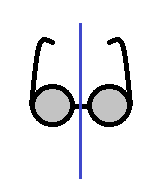(2) Lock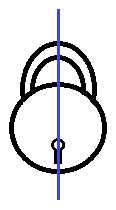(3) Glass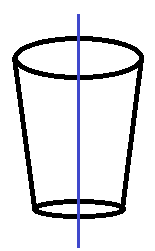(4) Ball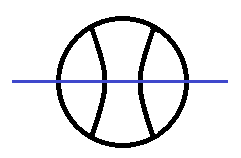(5) Pot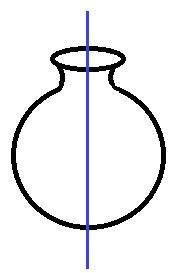Note: There can be more than one line of symmetry for one image or object. $1$ line symmetry – Image or object having one line of symmetry,$2$ lines symmetry – Image or object having two lines of symmetry, $3$ lines symmetry – Image or object having three lines of symmetry and Infinite lines – Some image not only have $1$ or $2$ lines of symmetry but infinite many lines pass through the center of the image.